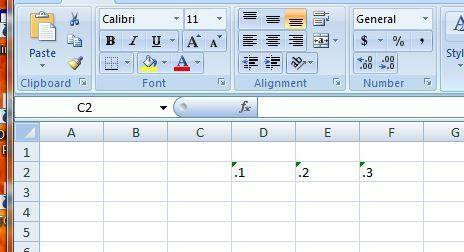# Excel data return

• Steven Ellet

#### Steven Ellet

If I want to lookup row 3 column "amz" and the end result will be side by side, how do i do that Example: a=.4 m=.91 z=.22
input; amz
output: .4.91.22

Don't know how you would do it in Excel formulas but here's how you do it in VBA (as an example)

I've put the kind of strings you show into 3 consecutive cells:Then created a VBA macro:
Code:
Sub string1()
Dim a As String
a = Cells(2, 4)
MsgBox Cells(2, 4) & Cells(2, 5) & Cells(2, 6)
End Sub

and the result is ".1.2.3"

If I want to lookup row 3 column "amz" and the end result will be side by side, how do i do that Example: a=.4 m=.91 z=.22
input; amz
"Column amz" is meaningless in Excel. The only thing I can think of that has any meaning is (R3,Ca)&(R3,Cm)&(R3,Cz) In these expressions R=row, C=column and "&" means "concatenation" (strings joined together). Post #2 gives a hint as to how to do the concatenation, but parsing the "amz" part is tricky.

Well if what I was hoping for won't work then how can I quickly pull data from multiple cells, even if I have a lot of data to pull?

Well if what I was hoping for won't work then how can I quickly pull data from multiple cells, even if I have a lot of data to pull?
One way: Structure your data as a database table and do queries. The trick lies in indexing the data...